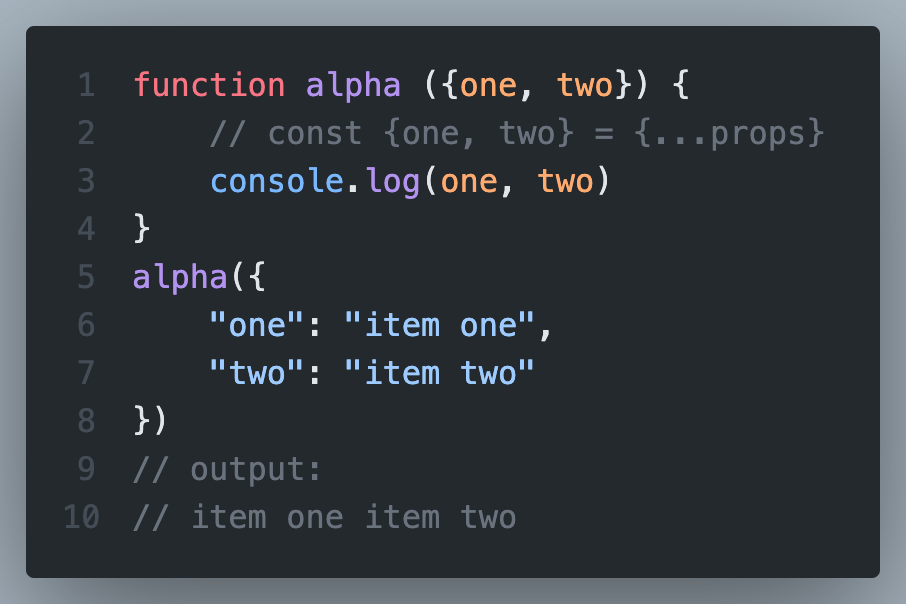Just another WordPress site# JavaScript Spread operator – arrays vs objectsJavaScript spread operator is the simple three dot notation which spreads the array or object for us.

For example:

``const {one, two} = {...props}``

However, there are some differences in how we use them with regards to arrays and objects.

## JavaScript spread operator for arrays

JavaScript spread operator for arrays is simple and straight forward. Check these examples:

``````function alpha (props) {
const [one, two] = [...props]
console.log(one, two)
}
alpha([
"item one",
"item two"
])
// output:
// item one item two``````

Here we simply spread the incoming props into two seperate const. Note that we’re using square brackets at all places, as we’re working with props as an array. So the const statement will also contain the square brackets.

Note that the names of the new variables in const line are not important. We can name them anything we want, and they will take the value based on the index position in the props array. So first var takes first value, second takes second and so on. This behaviour is different from object format props (discussed below).

## JavaScript Spread operator for Objects

Objects are defined slightly different. They also have a key, not just the value. So our code with props as an object will become:

``````function alpha (props) {
const {one, two} = {...props}
console.log(one, two)
}
alpha({
"one": "item one",
"two": "item two"
})
// output:
// item one item two``````

Notice how all square brackets are not changed to curly braces. The output still remains the same.

However, one more important thing, the keys being used to create the new variables in cost line should be same as the keys being sent inside props. It will give an error if we use a key which is not in them already. We do not have that limitation in array format, as it simply assigns the value to the new variables based on their index position, not name.

## Spreading the props into variables in function parameters

We can spread the incoming array or object to the function right within the function definition where it receives it. However, we can not just spread it and expect the passed keys to be names as variables automatically. So the code should be like this for array props:

``````function alpha ([one,two]) {
// const [one, two] = [...props]
console.log(one, two)
}
alpha([
"item one",
"item two"
])
// output:
// item one item two``````

And for object based props:

``````function alpha ({one, two}) {
// const {one, two} = {...props}
console.log(one, two)
}
alpha({
"one": "item one",
"two": "item two"
})
// output:
// item one item two``````

So we see, that the output of both the blocks is still the same.

Remember that once we spread the props (or any other parameter) like this, the code doesn’t have access to the parent props variable anymore, so we are not passing it inside the function anymore (instead passing in the variables created by spreading it).

Note that we can not simply spread the props in function parameters. So this won’t work:

``````function alpha ({...props}) {
// .... code here
}``````

I hope this helps you understand how you can use spread operator for both arrays and objects. You can use it regular javascript as well as in react js (or nextjs, nodejs, etc.).